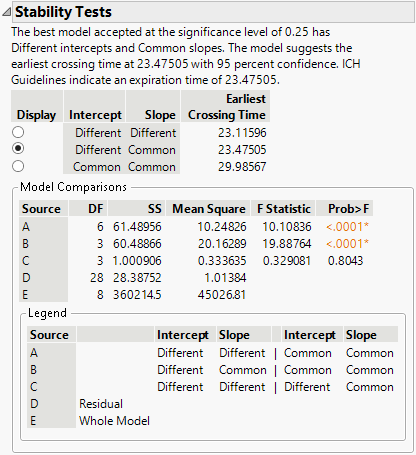Publication date: 08/13/2020

# Stability Analysis

Stability analysis is used in setting pharmaceutical product expiration dates. Three linear degradation models are fit, and an expiration date is estimated following ICH (ICH Q1E 2003) guidelines. The ICH guidelines are used for the general framework of determining if batches can be pooled. For specific implementation details, see the STAB macro and FDA guidelines in Chow (2007, Appendix B).

The three models are the following:

Model I

Uses different slopes and different intercepts for the batches.

Model II

Uses a common slope and different intercepts for the batches.

Model III

Uses a common slope and a common intercept for the batches.

The recommended model is determined by the following procedure:

1. Fit Model I with the time effect coming first in the model, followed by the batch effect, then the interaction. Using Type I (Sequential) sums-of-squares, test for equal slopes (Source C in the output).

If the p-value is less than 0.25, the slopes are assumed to be different across batches. The procedure stops and Model I is used to estimate the expiration date.

If the p-value is greater than or equal to 0.25, the slopes are assumed to be common across batches. The procedure continues to step 2.

2. If the conclusion from step 1 is common slopes, then test for equal intercepts using Type I (Sequential) sums-of-squares from Model II (Source B in the output).

If the p-value is less than 0.25, the intercepts are assumed to be different across batches, and Model II is used to estimate the expiration date.

If the p-value is greater than or equal to 0.25, the intercepts are assumed to be common across batches, and Model III is used to estimate the expiration date.

When Model I (different slopes and different intercepts) is used for estimating the expiration date, the MSE (mean squared error) is not pooled across batches. Prediction intervals are computed for each batch using individual mean squared errors, and the interval that crosses the specification limit first is used to estimate the expiration date.

Caution: The numbering of the models below the Residual Plot in the Degradation Data Analysis report does not necessarily correspond to the order of the models in the Stability Tests report. By default, Model 1 uses a pooled standard error and is not referenced in the Stability Tests report. Model 2 is equivalent to Model II in the Stability Tests report. Model 3 is equivalent to Model III in the Stability Tests report. Model 4 is equivalent to Model I in the Stability Tests report. If any other models are fit before or after requesting the Stability Test option, this model numbering equivalence might not hold.

### Example

Consider the Stability.jmp sample data table. The data consists of product concentration measurements on four batches. A concentration of 95 is considered the end of the product’s usefulness. Use the data to establish an expiration date for the new product.

To perform the stability analysis, do the following steps:

1. Select Help > Sample Data Library and open Reliability/Stability.jmp.

2. Select Analyze > Reliability and Survival > Degradation.

3. Select the Stability Test tab.

4. Select Concentration (mg/Kg) and click Y, Response.

5. Select Time and click Time.

6. Select Batch Number and click Label, System ID.

7. Enter 95 for the Lower Spec Limit.

8. Click OK.

Figure 7.24 Stability ModelsThe test for equal slopes has a p-value of 0.8043. Because this is larger than a significance level of 0.25, the test is not rejected, and you conclude the degradation slopes are equal between batches.

The test for equal intercepts and slopes has a p-value of <.0001. Because this is smaller than a significance level of 0.25, the test is rejected, and you conclude that the intercepts are different between batches.

Because the test for equal slopes was not rejected, and the test for equal intercepts was rejected, the chosen model is the one with Different Intercepts and Common Slope. This model is the one selected in the report, and gives an estimated expiration date of 23.475.